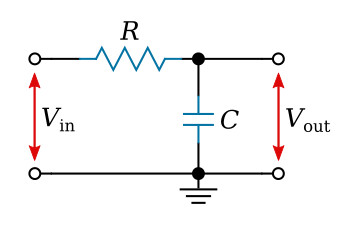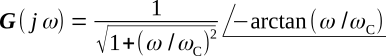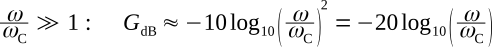Home   >   Textbooks   >   Basic Electronics   >   Filters   >   RC Passive Low-Pass Filter   >

Filters

# RC Passive Low-Pass Filter

A low-pass filter passes frequencies below a certain cutoff frequency and attenuates those beyond that frequency.RC low-pass filter.

The first circuit we shall analyze is that of an RC low-pass filter, as shown in the figure above. Before launching into a mathematical analysis, we can deduce some of the electrical properties by visual inspection of the circuit.

If the applied voltage is of very low frequency, the reactance of C will be very high compared with R, and C may be considered an open circuit. Therefore, at low frequencies the input voltage Vin will appear virtually unattenuated at the output. Hence, we have the name low-pass filter. As the input frequency increases, the reactance XC becomes smaller, causing the input to be increasingly attenuated. At an infinitely high frequency, XC = 0 and therefore the output voltage Vout = 0.

To analyze the circuit mathematically, we would use the voltage-divider relationship and writeWe shall, however, solve the ratio of Vout to Vin, since we generally wish to express the filter gain or loss. This ratio is called the transfer function. As a transfer function, we then havewhere ωC = 1/RC is the characteristic frequency.

The transfer function can be expressed in polar form## Frequency response

A most useful means of displaying the frequency characteristics of a filter is to plot the magnitude of the transfer function (amplitude characteristic) versus frequency on one curve and the phase characteristic as a separate curve but with the same frequency axis. The amplitude characteristic, which may vary over a wide range, can be conveniently plotted in terms of decibels. Curves with this type of display are known as Bode plots and find wide application in the analysis of AC circuits.

## Amplitude Characteristic

Consider first the amplitude characteristic (spectrum) corresponding to the equation above. This is the absolute value (magnitude) of the transfer function, orOn a decibel basis, this becomesLet us examine the equation above for very low and for very high frequencies. For low frequencies, we haveThus, the low-frequency behavior is essentially independent of frequency and can be represented by a horizontal straight line at 0 dB as in the figure below. The actual amplitude characteristic given by the transfer function is asymptotic to this straight line for small ω.Broken-line approximation and actual characteristic for the amplitude spectrum of the RC filter.

For the other extreme, we haveThis is of the form GdB = -20x, where x = log10 (ω/ωC). The straight line so defined is the high-frequency asymptote of the actual characteristic. The slope of the asymptote is dGdB/dx = -20; that is, when x increases one unit, GdB decreases by 20 dB. Butand so ω/ωC must increase by a factor of 10, or one decade, to make x increase one unit. Therefore, the slope of the high-frequency asymptote is -20 dB per decade. Some people prefer to use the octave (frequency ratio of 2:1). The corresponding slope is -6 dB per octave. The two straight-line asymptotes intersect at ω/ωC = 1, for then the amplitude characteristic has the value zero. The two asymptotes are shown dashed in the figure above. Their point of intersection, ω = ωC, in addition to being termed the characteristic frequency of the circuit, is also called the break point, or cutoff frequency. Together, the two asymptotes form a broken-line approximation to the actual characteristic. Depending upon the accuracy desired, neither line may be a sufficiently good approximation to the actual characteristic in the neighborhood of ω = ωC. It can be shown that the maximum error occurs at ω/ωC = 1 and is approximately 3 dB. Further more, an octave away from this point (at ω/ωC = 0.5 and ω/ωC = 2) the error is approximately 1 dB. From this, it is easy to sketch the actual amplitude characteristic with reasonable accuracy. The actual characteristic is shown by the solid line in the figure above. The low-pass characteristic of the circuit is easily seen in this figure.

## Phase Characteristic

Let us now consider the angle of the transfer function of the filter (sometimes called the phase spectrum), which isThe phase angle starts at zero for ω = 0 and approaches -π/2 radians at large ω. The phase characteristic can be approximated reasonably well by three straight-line segments, as shown in the figure below: a low-frequency approximation at 0 radians, a high-frequency approximation at -π/2 radians, and an intermediate-frequency approximation which is tangent to the curve at -π/4 radians. It can be shown that the middle segment intersects the low-frequency approximation at ω/ωC = 1/4.81 and intersects the high-frequency approximation at ω/ωC = 4.81. The actual characteristic is shown by the solid line in the figure.Broken-line approximation and actual characteristic for the phase spectrum of the RC filter.